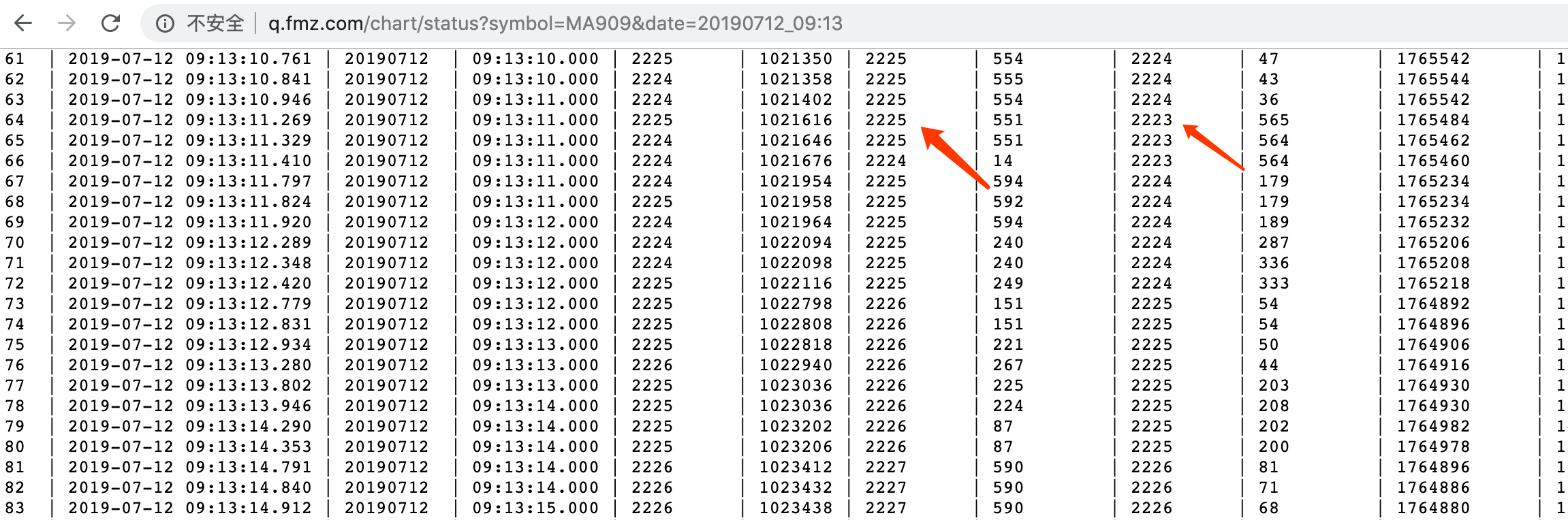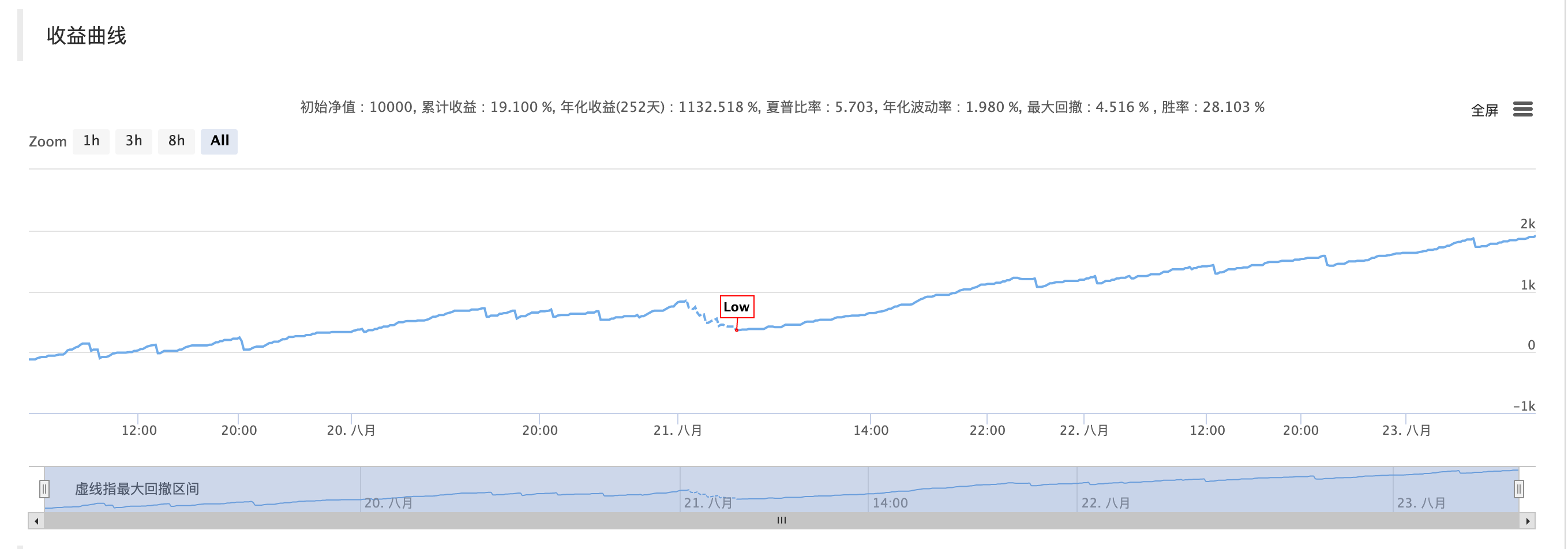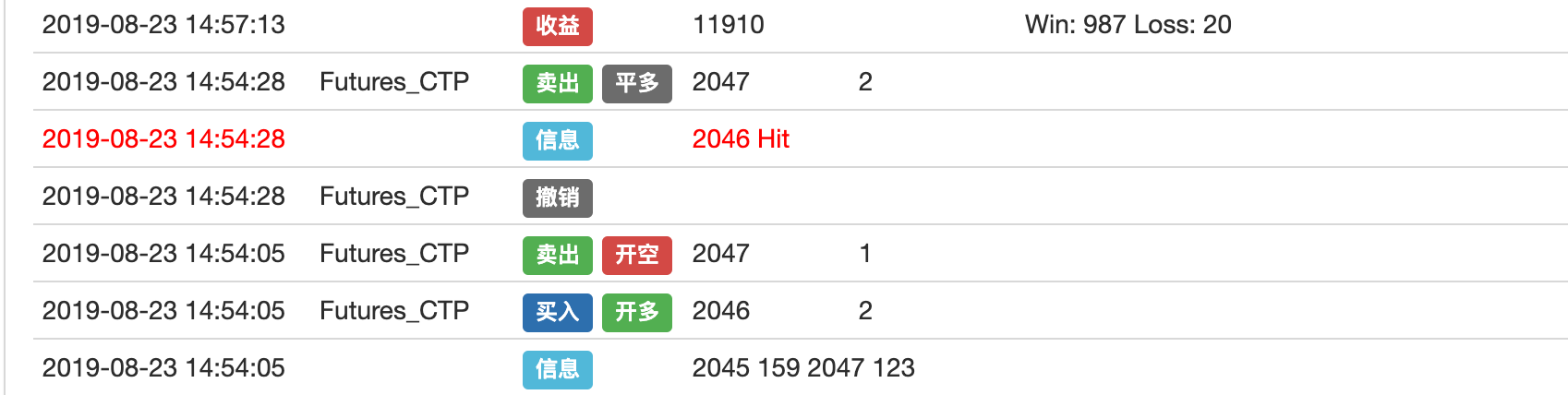# 什么是 Penny Jump 策略

Penny Jump 翻译成中文就是微量加价的意思，其原理是跟踪盘口买价和卖价，然后不停的根据盘口价格加上或减去微量价格挂单，很明显这是一个被动成交的挂单策略，属于卖方做市策略的一种。它的业务模式和商业逻辑是在交易所挂限价单进行双边交易以提供流动性。

# Penny Jump 策略实现# 策略源码

``````// 定义HFT类
class HFT {
public:
HFT() {
// 构造函数
}

int getTradingWeekDay() {
// 获取当前是星期几，用来判断是否为新的K线
}

State getState() {
// 获取订单数据
}

void stop() {
// 打印订单和持仓
}

bool Loop() {
// 策略逻辑和下单
}
};

// 主函数
void main() {
LogReset();  // 清除日志
SetErrorFilter("ready|timeout");  // 过滤错误信息
Log("Init OK");  // 打印日志
HFT hft;  // 创建HFT对象
while (hft.Loop());  // 进入无线循环模式
Log("Exit");  // 程序退出，打印日志
}
``````

``````// 定义全局枚举类型State
enum State {
STATE_NA,  // 储存订单状态
STATE_IDLE,  // 储存持仓状态
STATE_HOLD_LONG,  // 储存持多单方向
STATE_HOLD_SHORT,  // 储存持空单方向
};

// 定义全局浮点类型变量
typedef struct {
double bidPrice;  // 储存买价
double bidAmount;  // 储存买量
double askPrice;  // 储存卖价
double askAmount;  // 储存卖量
} Book;
``````

``````public:
// 构造函数
HFT() {
_tradingDay = getTradingWeekDay();
Log("current trading weekday", _tradingDay);
}

// 获取当前是星期几，用来判断是否为新的K线
int getTradingWeekDay() {
int seconds = Unix() + 28800;  // 获取时间戳
int hour = (seconds/3600)%24;  // 小时
int weekDay = (seconds/(60*60*24))%7+4;  // 星期
if (hour > 20) {
weekDay += 1;
}
return weekDay;
}

// 获取订单数据
State getState() {
auto orders = exchange.GetOrders();  // 获取所有订单
if (!orders.Valid || orders.size() == 2) {  // 如果没有订单或者订单数据的长度等于2
return STATE_NA;
}

bool foundCover = false;  // 临时变量，用来控制取消所有未成交的订单
// 遍历订单数组，取消所有未成交的订单
for (auto &order : orders) {
if (order.Id == _coverId) {
if ((order.Type == ORDER_TYPE_BUY && order.Price < _book.bidPrice - _toleratePrice) ||
(order.Type == ORDER_TYPE_SELL && order.Price > _book.askPrice + _toleratePrice)) {
exchange.CancelOrder(order.Id, "Cancel Cover Order");  // 根据订单ID取消订单
_countCancel++;
_countRetry++;
} else {
foundCover = true;
}
} else {
exchange.CancelOrder(order.Id);  // 根据订单ID取消订单
_countCancel++;
}
}
if (foundCover) {
return STATE_NA;
}

// 获取持仓数据
auto positions = exchange.GetPosition();  // 获取持仓数据
if (!positions.Valid) {  // 如果持仓数据为空
return STATE_NA;
}

// 遍历持仓数组，获取具体的持仓信息
for (auto &pos : positions) {
if (pos.ContractType == Symbol) {
_holdPrice = pos.Price;
_holdAmount = pos.Amount;
_holdType = pos.Type;
return pos.Type == PD_LONG || pos.Type == PD_LONG_YD ? STATE_HOLD_LONG : STATE_HOLD_SHORT;
}
}
return STATE_IDLE;
}

// 打印订单和持仓
void stop() {
Log(exchange.GetOrders());  // 打印订单
Log(exchange.GetPosition());  // 打印持仓
Log("Stop");
}
``````

``````// 策略逻辑和下单
bool Loop() {
if (exchange.IO("status") == 0) {  // 如果CTP交易和行情服务器已经连接
LogStatus(_D(), "Server not connect ....");  // 打印信息到状态栏
Sleep(1000);  // 休眠1秒
return true;
}

if (_initBalance == 0) {
_initBalance = _C(exchange.GetAccount).Balance;  // 获取账户余额
}

auto day = getTradingWeekDay();  // 获取星期数
if (day != _tradingDay) {
_tradingDay = day;
_countCancel = 0;
}

// 设置期货合约类型，并获取合约具体信息
if (_ct.is_null()) {
Log(_D(), "subscribe", Symbol);  // 打印日志
_ct = exchange.SetContractType(Symbol);  // 设置期货合约类型
if (!_ct.is_null()) {
auto obj = _ct["Commodity"]["CommodityTickSize"];
int volumeMultiple = 1;
if (obj.is_null()) { // CTP
obj = _ct["PriceTick"];
volumeMultiple = _ct["VolumeMultiple"];
_exchangeId = _ct["ExchangeID"];
} else { // Esunny
volumeMultiple = _ct["Commodity"]["ContractSize"];
_exchangeId = _ct["Commodity"]["ExchangeNo"];
}
if (obj.is_null() || obj <= 0) {
Panic("PriceTick not found");
}
if (_priceTick < 1) {
exchange.SetPrecision(1, 0);  // 设置价格与品种下单量的小数位精度, 设置后会自动截断
}
_priceTick = double(obj);
_toleratePrice = _priceTick * TolerateTick;
_ins = _ct["InstrumentID"];
Log(_ins, _exchangeId, "PriceTick:", _priceTick, "VolumeMultiple:", volumeMultiple);  // 打印日志
}
Sleep(1000);  // 休眠1秒
return true;
}

// 检查订单和头寸以设置状态
auto depth = exchange.GetDepth();  // 获取深度数据
if (!depth.Valid) {  // 如果没有获取到深度数据
LogStatus(_D(), "Market not ready");  // 打印状态信息
Sleep(1000);  // 休眠1秒
return true;
}
_countTick++;
_preBook = _book;
_book.bidPrice = depth.Bids.Price;  // 买一价
_book.bidAmount = depth.Bids.Amount;  // 买一量
_book.askPrice = depth.Asks.Price;  // 卖一价
_book.askAmount = depth.Asks.Amount;  // 卖一量
// 判断盘口数据赋值状态
if (_preBook.bidAmount == 0) {
return true;
}
auto st = getState();  // 获取订单数据

// 打印盘口数据到状态栏
LogStatus(_D(), _ins, "State:", st,
"Ask:", depth.Asks.Price, depth.Asks.Amount,
"Bid:", depth.Bids.Price, depth.Bids.Amount,
"Cancel:", _countCancel,
"Tick:", _countTick);
}
``````

``````bool forceCover = _countRetry >= _retryMax;  // 布尔值，用来控制平仓次数
if (st == STATE_IDLE) {  // 如果无持仓
if (_holdAmount > 0) {
if (_countRetry > 0) {
_countLoss++;  // 失败计数
} else {
_countWin++;  // 成功技术
}
auto account = exchange.GetAccount();  // 获取账户信息
if (account.Valid) {  // 如果获取到账户信息
LogProfit(_N(account.Balance+account.FrozenBalance-_initBalance, 2), "Win:", _countWin, "Loss:", _countLoss);  // 记录盈利值
}
}
_countRetry = 0;
_holdAmount = 0;

// 判断撤单状态
if (_countCancel > _cancelMax) {
Log("Cancel Exceed", _countCancel);  // 打印日志
return false;
}

bool canDo = false;  // 临时变量
if (abs(_book.bidPrice - _book.askPrice) > _priceTick * 1) {  // 如果当前盘口买价与卖价之间是否有2跳以上的空间
canDo = true;
}
if (!canDo) {
return true;
}

auto bidPrice = depth.Bids.Price;  // 买一价
auto askPrice = depth.Asks.Price;  // 卖一价
auto bidAmount = 1.0;
auto askAmount = 1.0;

if (_preBook.bidPrice > _book.bidPrice && _book.askAmount < _book.bidAmount) {  // 如果之前的买价大于当前的买价，并且当前的卖量小于买量
bidPrice += _priceTick;  // 设置开多单价格
bidAmount = 2;  // 设置开多单量
} else if (_preBook.askPrice < _book.askPrice && _book.bidAmount < _book.askAmount) {  // 如果之前的卖价小于当前的卖价，并且当前买量小于卖量
askPrice -= _priceTick;  // 设置开空单价格
askAmount = 2;  // 设置开空单量
} else {
return true;
}
Log(_book.bidPrice, _book.bidAmount, _book.askPrice, _book.askAmount);  // 打印当前盘口数据
exchange.SetDirection("buy");  // 设置下单类型为多头
exchange.Buy(bidPrice, bidAmount);  // 多头买入开仓
exchange.SetDirection("sell");  // 设置下单类型为空头
exchange.Sell(askPrice, askAmount);  // 空头卖出开仓
}
``````

``````else if (st == STATE_HOLD_LONG) {  // 如果持多单
exchange.SetDirection((_holdType == PD_LONG && _exchangeId == "SHFE") ? "closebuy_today" : "closebuy");  // 设置下单类型，及平昨仓或平今仓
auto sellPrice = depth.Asks.Price;  // 获取卖一价
if (sellPrice > _holdPrice) {  // 如果当前卖一价大于多单开仓价格
Log(_holdPrice, "Hit #ff0000");  // 打印多单开仓价格
sellPrice = _holdPrice + ProfitTick;  // 设置平多单价格
} else if (sellPrice < _holdPrice) {  // 如果当前卖一价小于多单开仓价格
forceCover = true;
}
if (forceCover) {
Log("StopLoss");
}
_coverId = exchange.Sell(forceCover ? depth.Bids.Price : sellPrice, _holdAmount);  // 平多单
if (!_coverId.Valid) {
return false;
}
}
``````

``````else if (st == STATE_HOLD_SHORT) {  // 如果持空单
exchange.SetDirection((_holdType == PD_SHORT && _exchangeId == "SHFE") ? "closesell_today" : "closesell");  // 设置下单类型，及平昨仓或平今仓
auto buyPrice = depth.Bids.Price;  // 获取买一价
if (buyPrice < _holdPrice) {  // 如果当前买一价小于空单开仓价格
Log(_holdPrice, "Hit #ff0000");  // 打印日志
buyPrice = _holdPrice - ProfitTick;  // 设置平空单价格
} else if (buyPrice > _holdPrice) {  // 如果当前买一价大于空单开仓价格
forceCover = true;
}
if (forceCover) {
Log("StopLoss");
}
_coverId = exchange.Buy(forceCover ? depth.Asks.Price : buyPrice, _holdAmount);  // 平空单
if (!_coverId.Valid) {
return false;
}
}
``````

# 回测结果# 交易逻辑# 关于我们

##### 相关下载
###### 登录后评论
Copyright © 2019 宽客在线